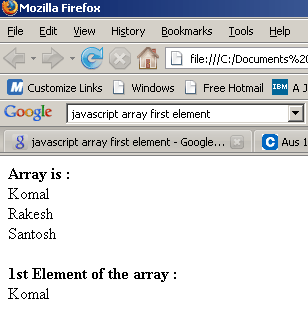Tutorials

# JavaScript array first element

In this Tutorial we want to describe you a code that makes you easy to understand in array first element example.

In this Tutorial we want to describe you a code that makes you easy to understand in array first element example.

# JavaScript array first element

In this Tutorial we want to describe you a code that makes you easy to understand in array first element example. For this we are using Java Scripting language This code show a page on loading invokes a function display. This function  include a variable array that is used to instantiate an array object. The array object hold the various element .The for loop executes and run the script till the variable i is less than 3.The document. write print the element from  array index-.The document. write[array(0)] print the 1st element hold by an array object in index i.e Komal.

First Element.java

 ```      First Element                      ```

Output